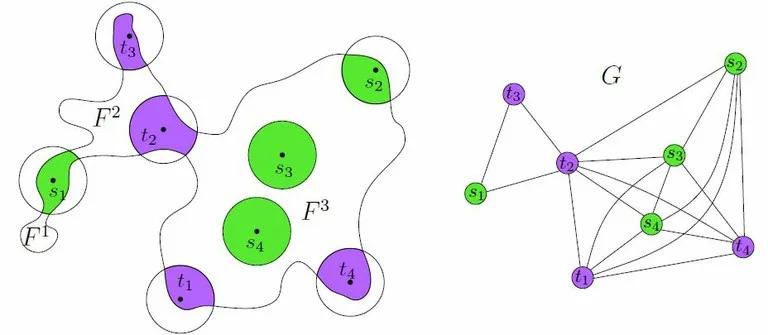# Efficient Multi-Robot Motion Planning for Unlabeled Discs in Simple Polygons

## Abstract

We consider the following motion-planning problem: we are given m unit discs in a simple polygon with n vertices, each at their own start position, and we want to move the discs to a given set of m target positions. Contrary to the standard (labeled) version of the problem, each disc is allowed to be moved to any target position, as long as in the end every target position is occupied. We show that this unlabeled version of the problem can be solved in O(mn+m^2) time, assuming that the start and target positions are at least some minimal distance from each other. This is in sharp contrast to the standard (labeled) and more general multi-robot motion planning problem for discs moving in a simple polygon, which is known to be strongly NP-hard.Figure: Illustration of the technique described in the paper. The free space is decomposed into regions and modeled as a graph.In order to solve the unlabeled problem we solve a special case of a pebble problem, which is a discrete version of the multi-robotproblem. A solution to the pebble problem can be transformed into a set of collision free paths for the continuous unlabeled problem.

• Aviv Adler, Mark de Berg, Dan Halperin and Kiril Solovey (*alphabetical order)
Efficient Multi-Robot Motion Planning for Unlabeled Discs in Simple Polygons
Transactions on Automation Science and Engineering (T-ASE), 2015 [link].
In Proceedings of the 11th International Workshop on the Algorithmic Foundations of Robotics (WAFR)2014 [link].